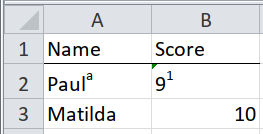## 5.5 Superscript symbolsThis is pernicious. What was Paula’s score, in the table below?

``````path <- system.file("extdata", "worked-examples.xlsx", package = "unpivotr")
``````## # A tibble: 2 x 2
##   Name    Score
##   <chr>   <chr>
## 1 Paula   91
## 2 Matilda 10``````

The answer is, it’s not Paula, it’s Paul (superscript ‘a’), who scored 9 (superscript ‘1’).

This sort of thing is difficult to spot. There’s a clue in the ‘Score’ column, which has been coerced to character so that the author could enter the superscript ‘1’ (Excel doesn’t allow superscripts in numeric cells), But it would be easy to interpret that as an accident of translation, and simply coerce back to numeric with `as.integer()`.

With tidyxl, you can count the rows of each element of the `character_formatted` column to identify cells that have in-cell formatting.

``````xlsx_cells(path, sheet = "superscript symbols") %>%
dplyr::filter(data_type == "character") %>%
dplyr::filter(map_int(character_formatted, nrow) != 1) %>%
select(row, col, character)``````
``````## # A tibble: 2 x 3
##     row   col character
##   <int> <int> <chr>
## 1     2     1 Paula
## 2     2     2 91``````

The values and symbols can then be separated by assuming the value is the first string, and the symbol is the second.

``````xlsx_cells(path, sheet = "superscript symbols") %>%
mutate(character = map_chr(character_formatted,
~ ifelse(is.null(.x), character, .x\$character)),
symbol = map_chr(character_formatted,
~ ifelse(is.null(.x), NA, .x\$character)),
numeric = if_else(row > 1 & col == 2 & data_type == "character",
as.numeric(character),
numeric),
character = if_else(is.na(numeric), character, NA_character_)) %>%
select(row, col, numeric, character, symbol)``````
``````## Warning in if_else(row > 1 & col == 2 & data_type == "character", as.numeric(character), : NAs
## introduced by coercion``````
``````## # A tibble: 6 x 5
##     row   col numeric character symbol
##   <int> <int>   <dbl> <chr>     <chr>
## 1     1     1      NA Name      <NA>
## 2     1     2      NA Score     <NA>
## 3     2     1      NA Paul      a
## 4     2     2       9 <NA>      1
## 5     3     1      NA Matilda   <NA>
## 6     3     2      10 <NA>      <NA>``````